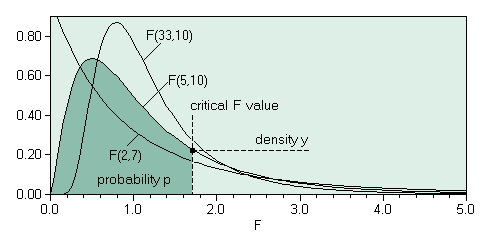The SDL Component Suite is an industry leading collection of components supporting scientific and engineering computing. Please visit the SDL Web site for more information....## FDistriIntegralUnit: SDL_statis Class: none Declaration: function FDistriIntegral (F: double; df1, df2: integer): double;

The function FDistriIntegral returns the integral of the F-distribution between zero and the value of the parameter F. The parameters df1 and df2 define the degrees of freedom and must be greater or equal to 1. The returned value may vary between 0.0 and 1.0.Hint 1: The calculated value is exact to about 8 decimal places.

 Hint 2: The function FDistriIntegral calculates the integral from 0.0 to the F value. This differs from the convention of how the integral of the F distribution is used in many statistical packages, which tabulate the integral between +infinity and the F value. Thus you have to use 1-FDistriIntegral to get comparable results. For example, in order to calculate the function FDIST(x,df1,df2) of Microsofts Excel® you have to use 1-FDistriIntegral(x,df1,df2).

 Example: This function is used in the following example program (see http://www.lohninger.com/examples.html for downloading the code): fdistri

Last Update: 2020-Aug-12